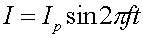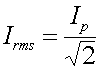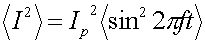R.M.S. - what it means An AC circuit has current with the behavior of sine/cosine waves.The peak current Ip gives one way to reference the strength of the current. The r.m.s. valueis another. The r.m.s. value is a sort of averaging, as the name root-mean-square implies. If one takes the average over time of the squared current, one gets the peak current squared times the average of the sine function:The average of sin2 is a half, thus the square root of the average squared current comes out to be 1/sqrt(2) times the peak. This is somewhat useful when considering such quantities as the power in a resistor which go as I(t)2R, thus if one uses the r.m.s. value of the current one obtains the average power. Many quantities are quadratic in the current. When one refers to the 110 V outlets in American homes, one is actually quoting the r.m.s. value.